# Find the area under the graph of f over the interval [0,4]. f(x) = x^2 for x< or equal to 2 20...

Find the area under the graph of f over the interval [0,4].

f(x) = x^2 for x< or equal to 2

20-4x for x>2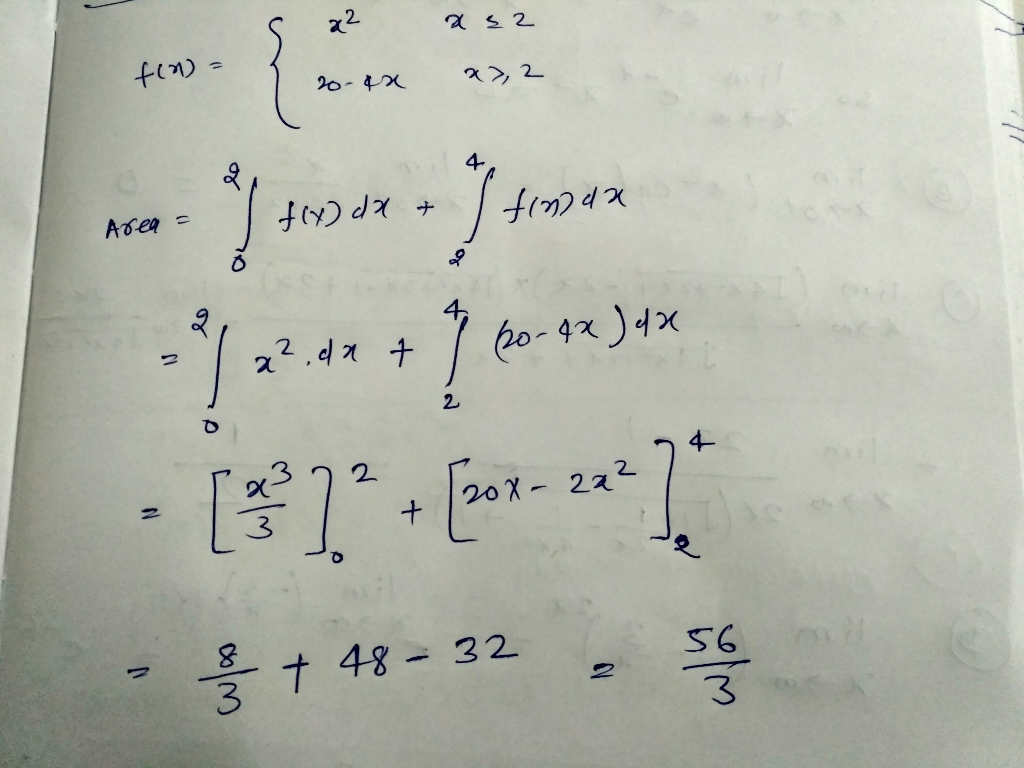##### Add Answer of: Find the area under the graph of f over the interval [0,4]. f(x) = x^2 for x< or equal to 2 20...
Similar Homework Help Questions
• ### under the Curve 2. Let y e2". a) Using 4 rectangles of equal width (Δ 1)and the rightendpoint of the subinterval for the height of the rectangle, estimate the area under the curve on the interval...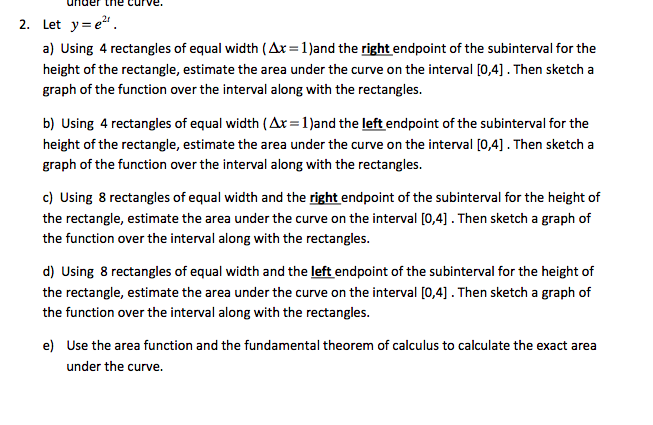under the Curve 2. Let y e2". a) Using 4 rectangles of equal width (Δ 1)and the rightendpoint of the subinterval for the height of the rectangle, estimate the area under the curve on the interval [0,4]. Then sketch a graph of the function over the interval along with the rectangles. b) Using 4 rectangles of equal width (Ax 1)and the left endpoint of the subinterval for the height of the rectangle, estimate the area under the curve on the...

• ### Estimate the area of the region bounded by the graph of f(x)-x + 2 and the x-axis on [0,4] in the following ways a. Divide [0,4] into n = 4 subintervals and approximate the area of the region using...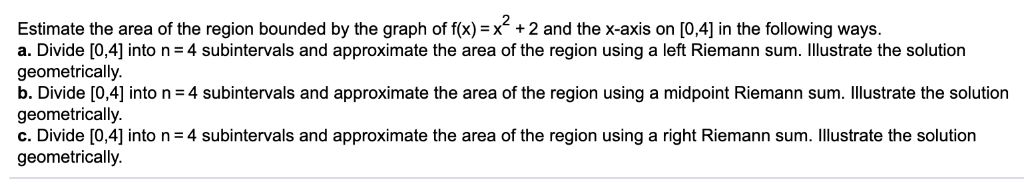Estimate the area of the region bounded by the graph of f(x)-x + 2 and the x-axis on [0,4] in the following ways a. Divide [0,4] into n = 4 subintervals and approximate the area of the region using a left Riemann sum. Illustrate the solution geometrically. b. Divide [0,4] into n = 4 subintervals and approximate the area of the region using a midpoint Riemann sum· illustrate the solution geometrically. C. Divide  into n = 4 subintervals and...

• ### The area under a graph

Book: Calculus and its applications 9th edition Bittinger and EllenbogenChapter 4.1 Problem 24a) Approximate the area under the graph of f(x)=x^2+1 over the interval [0,5] by computing the area of each rectangle and then the adding. Problem a graph has pointsat 0,1,2,3,4.. The graph has an f(4)b) Approximate the area under the graph of f(x)=x^2+1 over the interval [0,5] by computing the area of each rectangle and then adding. Compare your answer to that ofpart (a). Problem b graph has...

• ### Area under the Graph 4.1.7

Approximate the area under the graph of f(x) = 0.02x^\$ -2.25^2 +91 over the interval [6,10] by dividing the interval into 4 subintervals. Use the left endpoint of eachsubinterval.a) The area under the graph of f(x)= 0.02x^4 -2.25x^2 +91 over the interval [6,10] is approximately __________________>(SIMPLIFY YOUR ANSWER. TYPE AN INTERGER OR DECIMAL)

• ### full steps and how to solve please 1. Let y-x'. a) Using 4 rectangles of equal width (Ar-2 )and the right endpoi...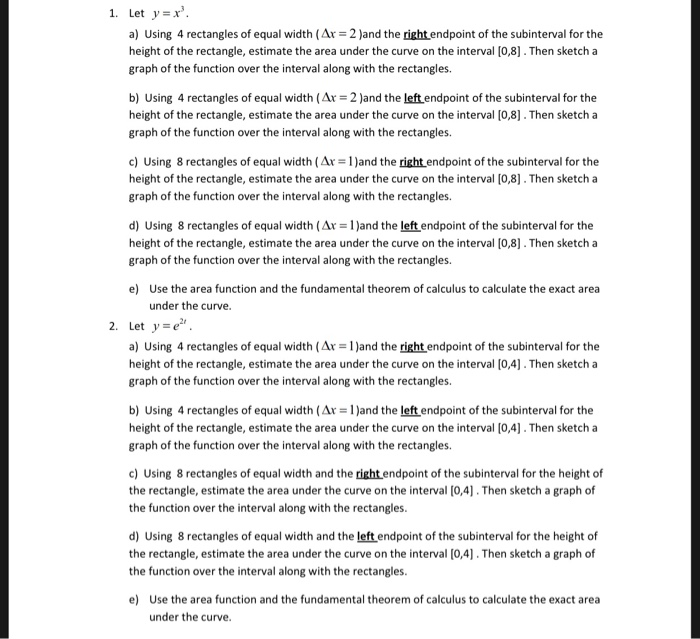full steps and how to solve please 1. Let y-x'. a) Using 4 rectangles of equal width (Ar-2 )and the right endpoint of the subinterval for the height of the rectangle, estimate the area under the curve on the interval [0,8. Then sketch a graph of the function over the interval along with the rectangles. b) Using 4 rectangles of equal width (Ax 2 and the left endpoint of the subinterval for the height of the rectangle, estimate the area...

• ### Find area of region

Find the area of the region under the graph of the function f on the interval [2, 7].f(x)= 4x-2

• ### 2. (Section 4.2) Given f(x)-x on the interval [0,4], complete the following (a) Verify that the f...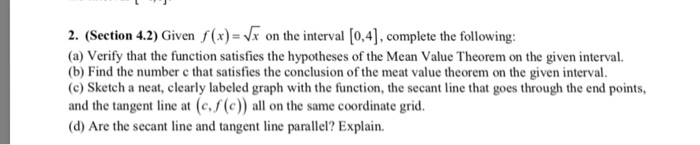2. (Section 4.2) Given f(x)-x on the interval [0,4], complete the following (a) Verify that the function satisfies the hypotheses of the Mean Value Theorem on the given interval. b) Find the number c that satisfies the conclusion of the meat value theorem on the given interval. (c) Sketch a neat, clearly labeled graph with the function, the secant line that goes through the end points, and the tangent line at (c./(c)) all on the same coordinate grid (d) Are...

• ### Area under the graph

Find an approximation of the area of the region R under the graph of the function f on the interval [1, 3]. Use n = 4 subintervals. Choose the representative points tobe the right endpoints of the subintervals.f (x)= 2/x

• ### Estimate the area under the curve f(x)=x^2-4x+5 on [1,3]

Estimate the area under the curve f(x)=x^2-4x+5 on [1,3]. Darw the graph and the midpoint rectangles using 8 partitions. Show how to calculate the estimated area by finding the sum of areas of the rectangles. Find the actual area under the curve on [1,3] using a definite integral.

• ### Find the area under the graph to the x axis from -2 to 2 for

Find the area under the graph to the x axis from -2 to 2 forf(x) = (4 - x2)^(1/2) . Round to two decimal places.

Need Online Homework Help?